|

# 聚焦​装饰行业大事件:广东御鼎国际集团五大品牌重组发布会召开

2019年12月9日，“御众之力·鼎创未来”五大品牌重组发布会于佛山美居国际建材中心隆重召开，一个全新的“大型装企管理服务母体平台”——广东御鼎管理咨询有限公司面世。这艘实力雄厚、规模庞大的“饰界航母”一下子聚焦了全行业的目光。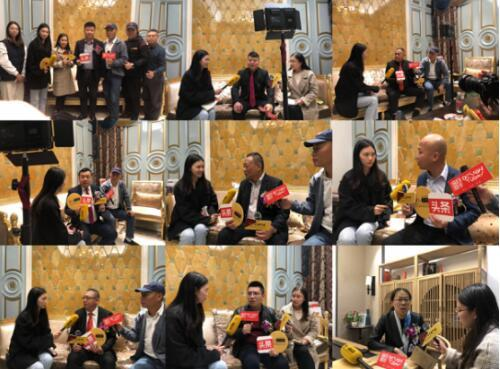纵观中国家装行业近几年沉浮，高速发展的同时伴随着激烈竞争，使得行业乱象依然存在：低端伪劣的装修设计服务、低价劣质整装工程、只打价格战不重质量、不诚信经营装企相继倒闭等，灾难重重，令消费者陷入误区，使消费者对装修公司产生普遍不信任心理，如何解决装修痛点、优化服务能力，让家庭装修变得轻松，让客户放心，成了当今装饰企业必要解决的问题。也是提高客户满意度的关键。在激烈的市场竞争下，如今不再是一个单打独斗的时代，而是联盟共赢的时代！未来的发展趋势必定是“资源整合，合作共赢”，广东御鼎创始人刘金霖跟进时代，抓住趋势，通过整理、整顿、整合，打造出一个强大有力的装企管理服务平台，打破行业格局，凝练出厚实的企业根基，有效的资源整合可以促进企业发展，增强企业的整体实力的同时，推动行业的良性发展。

五大品牌重组，重新赋能

为了助力装企的转型升级，提升服务竞争力，创造合理的利润空间支撑高品质的装修服务，做真正有品质的家装，真正向消费者传递高品质的居家生活体验，广东御鼎将美星装饰、托美尔装饰、春满人间装饰、新居缘装饰与南国鼎峰装饰五大品牌进行重组。其中，这五大品牌都是深耕经营近二十载的知名连锁品牌：

广东美星装饰成立20余载，旗下拥有两百多家分公司；广东春满人间装饰通过近20年时间发展了一百多家分公司；托美尔装饰作为装饰行业历史最悠久的行业创新者之一，拥有将近100家分公司；拥有近20年发展历史的新居缘装饰拥有近一百家分公司，而经营十余载的南国鼎峰装饰已实现三百多家分公司的发展目标。广东美星装饰成立于2000年，本着“精益求精，源于美星”的服务理念，发展至今，已在全国拥有两百七十多家分公司；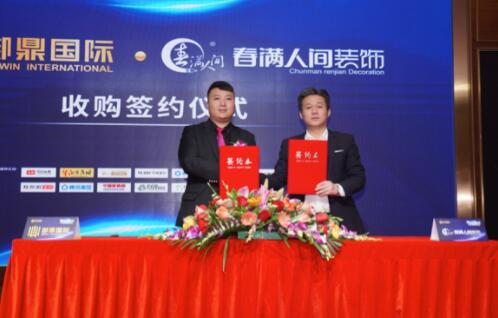广东春满人间装饰成立于2001年，秉承“一心一意，缔造和谐人居生活”的服务理念，通过近20年时间在全国发展了一百五十多家分公司；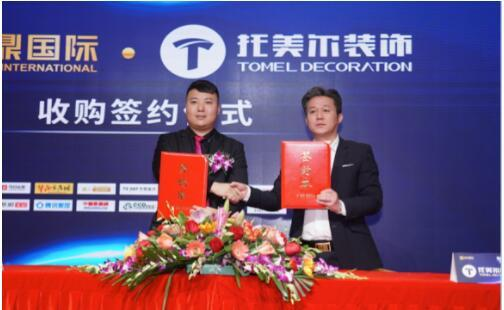广东托美尔装饰成立于1997年，作为装饰行业历史最悠久的行业创新者之一，以“品质人居，托起幸福生活”的服务理念，发展至今，在全国拥有一百多家分公司；广东南国鼎峰装饰坚持“环保家装就选南国鼎峰”服务理念，专注品质家装十余载，发展至今已实现全国三百多家分公司的发展目标。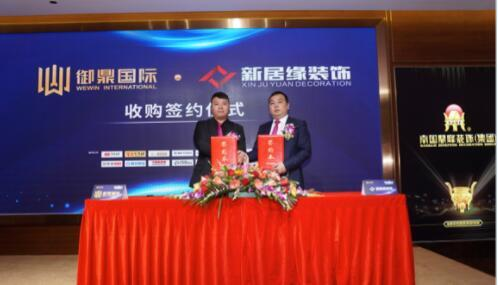广东新居缘装饰成立于2001年，以“全案高端定制，智享健康生活”服务理念，通过近20年时间，在全国发展近一百家分公司。

此次五大品牌重组，形成辐射区域涵盖八百城的大型装企管理服务母体平台——广东御鼎管理咨询有限公司。同时，在2019“御众之力，鼎创未来”发布会上，广东御鼎五大品牌与CCTV进行签约，荣登央视，正式向饰界发出最强音，整合五大品牌优势资源，提升服务竞争力，重建组织架构，重新赋能，以强大的本地化服务能力服务全国客户。

布局千城万店，创造企业规模经济效益

作为国内全新最大的装企母体平台，广东御鼎旗下拥有800多家装企分公司渠道，形成巨大的企业规模优势和规模影响力，并保持每年规模不断持续壮大的发展趋势；本地化服务能力提升并不断加强，从而具备更强的招商能力。广东御鼎创始人刘金霖在发布会上表示，广东御鼎平台将以“战略合作、强核多渠、资源共享、互惠互利”的共赢原则，拟建“品牌运营管理中心”、“战略企划部”、“电商网络技术部”、“工程督导中心”、“商学院”、“供应链服务部”、“法务部”等职能部门，以“品牌强化输出、商学院输出、运营管理模式输出、软装固装输出、辅材基装供应链输出、流量输出、营销工具输出、电商盘口技术输出、营销活动策划输出、财务管理输出、天使管理流程输出”为强大核心的商业模式提升服务竞争力，为装企创造更大的产值，为消费者打造高品质美好居家生活！在如今行业呈现出“大行业，小企业”的分散市场现状，而广东御鼎以“大平台，多渠道”的企业布局，抢占更大市场份额，满足市场需求，未来实现更大的规模经济效益。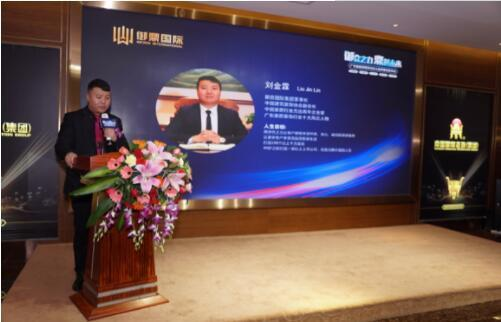为了打造装企高效的核心管理运营团队，实现人才输出，五大品牌重组后广东御鼎将组建商学院，与更多培训机构达成战略合作，共同研发装企运营新模式，建立一支真正高效的核心管理运营团队，为全国装企输送人才，提升服务能力，增强竞争实力，向终端输送更优质的装修服务。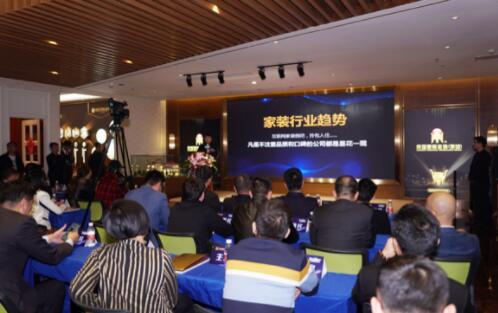广东御鼎将组建共享供应链平台，为渠道提供更具性价比的产品，与定制、建材、软装等生产型企业，共享产品及渠道。集团与全球各大一线材料品牌形成战略合作模式，汇集全球多家材料供应商，建立同步欧洲环保系数的主材及辅材选品标准体系，从辅材到主材到软装配饰无缝对接，形成完善的生态材料供应链，并以集采模式去中间环节化，让客户真正地享受到优于市场价格与品质的家装材料，同时让各大材料供应商创造了更大的利润空间，达成共赢。回归本质，将高品质家装进行到底

在风起云涌、竞争激烈的家装市场中，广东御鼎创始人刘金霖在不断创新改革家装商业模式的同时，始终坚守初心，立足原点，回归本质，将高品质家装进行到底。此次特邀出席广东御鼎发布会的中国装修教父、艺邦集团创始人余静赣余工，对广东御鼎的经营理念与发展战略表示深度的认同与支持，同时感恩众多来自于中国装饰之乡“武宁”的装修人对于装修事业的坚持与发展，并且相信广东御鼎能够带领五大品牌全国装企迈进成功的道路，为国人创造更高品质的人居环境。未来趋势，泛家居行业的融合

在此次发布会就“如何看待此次五大品牌重组与未来家装行业趋势”的主题座谈会上，行业大咖纷纷发表了各自独到的见解与想法，但就未来的趋势必定是“强强融合，合作共赢”达成共识。广东御鼎将秉承“共创、共享、共赢”的理念，整合上下游供应链，形成“品牌强化、服务强化、资源优化、品质优化、模式创新、技术创新”的融合特质，重建泛家居生态。未来，在更加激烈的家装竞争市场下，广东御鼎将继续整合全国更多的优质企业，与更多志同道合的企业合伙人汇聚资源和资金，聚集更多优质人才，创新研发新技术，优化服务模式，去不断创新、升级企业平台，全方位提升自身竞争力，提供给消费者和市场一个全新的服务和形象，共筑家装美好新未来！

`声明：本文由入驻焦点开放平台的作者撰写，除焦点官方账号外，观点仅代表作者本人，不代表焦点立场错误信息举报电话： 400-099-0099，邮箱：jubao@vip.sohu.com，或点此进行意见反馈，或点此进行举报投诉。`A B C D E F G H J K L M N P Q R S T W X Y Z
A - B - C - D - E
• A
• 鞍山
• 安庆
• 安阳
• 安顺
• 安康
• 澳门
• B
• 北京
• 保定
• 包头
• 巴彦淖尔
• 本溪
• 蚌埠
• 亳州
• 滨州
• 北海
• 百色
• 巴中
• 毕节
• 保山
• 宝鸡
• 白银
• 巴州
• C
• 承德
• 沧州
• 长治
• 赤峰
• 朝阳
• 长春
• 常州
• 滁州
• 池州
• 长沙
• 常德
• 郴州
• 潮州
• 崇左
• 重庆
• 成都
• 楚雄
• 昌都
• 慈溪
• 常熟
• D
• 大同
• 大连
• 丹东
• 大庆
• 东营
• 德州
• 东莞
• 德阳
• 达州
• 大理
• 德宏
• 定西
• 儋州
• 东平
• E
• 鄂尔多斯
• 鄂州
• 恩施
F - G - H - I - J
• F
• 抚顺
• 阜新
• 阜阳
• 福州
• 抚州
• 佛山
• 防城港
• G
• 赣州
• 广州
• 桂林
• 贵港
• 广元
• 广安
• 贵阳
• 固原
• H
• 邯郸
• 衡水
• 呼和浩特
• 呼伦贝尔
• 葫芦岛
• 哈尔滨
• 黑河
• 淮安
• 杭州
• 湖州
• 合肥
• 淮南
• 淮北
• 黄山
• 菏泽
• 鹤壁
• 黄石
• 黄冈
• 衡阳
• 怀化
• 惠州
• 河源
• 贺州
• 河池
• 海口
• 红河
• 汉中
• 海东
• I
• J
• 晋中
• 锦州
• 吉林
• 鸡西
• 佳木斯
• 嘉兴
• 金华
• 景德镇
• 九江
• 吉安
• 济南
• 济宁
• 焦作
• 荆门
• 荆州
• 江门
• 揭阳
• 金昌
• 酒泉
• 嘉峪关
K - L - M - N - P
• K
• 开封
• 昆明
• 昆山
• L
• 廊坊
• 临汾
• 辽阳
• 连云港
• 丽水
• 六安
• 龙岩
• 莱芜
• 临沂
• 聊城
• 洛阳
• 漯河
• 娄底
• 柳州
• 来宾
• 泸州
• 乐山
• 六盘水
• 丽江
• 临沧
• 拉萨
• 林芝
• 兰州
• 陇南
• M
• 牡丹江
• 马鞍山
• 茂名
• 梅州
• 绵阳
• 眉山
• N
• 南京
• 南通
• 宁波
• 南平
• 宁德
• 南昌
• 南阳
• 南宁
• 内江
• 南充
• P
• 盘锦
• 莆田
• 平顶山
• 濮阳
• 攀枝花
• 普洱
• 平凉
Q - R - S - T - W
• Q
• 秦皇岛
• 齐齐哈尔
• 衢州
• 泉州
• 青岛
• 清远
• 钦州
• 黔南
• 曲靖
• 庆阳
• R
• 日照
• 日喀则
• S
• 石家庄
• 沈阳
• 双鸭山
• 绥化
• 上海
• 苏州
• 宿迁
• 绍兴
• 宿州
• 三明
• 上饶
• 三门峡
• 商丘
• 十堰
• 随州
• 邵阳
• 韶关
• 深圳
• 汕头
• 汕尾
• 三亚
• 三沙
• 遂宁
• 山南
• 商洛
• 石嘴山
• T
• 天津
• 唐山
• 太原
• 通辽
• 铁岭
• 泰州
• 台州
• 铜陵
• 泰安
• 铜仁
• 铜川
• 天水
• 天门
• W
• 乌海
• 乌兰察布
• 无锡
• 温州
• 芜湖
• 潍坊
• 威海
• 武汉
• 梧州
• 渭南
• 武威
• 吴忠
• 乌鲁木齐
X - Y - Z
• X
• 邢台
• 徐州
• 宣城
• 厦门
• 新乡
• 许昌
• 信阳
• 襄阳
• 孝感
• 咸宁
• 湘潭
• 湘西
• 西双版纳
• 西安
• 咸阳
• 西宁
• 仙桃
• 西昌
• Y
• 运城
• 营口
• 盐城
• 扬州
• 鹰潭
• 宜春
• 烟台
• 宜昌
• 岳阳
• 益阳
• 永州
• 阳江
• 云浮
• 玉林
• 宜宾
• 雅安
• 玉溪
• 延安
• 榆林
• 银川
• Z
• 张家口
• 镇江
• 舟山
• 漳州
• 淄博
• 枣庄
• 郑州
• 周口
• 驻马店
• 株洲
• 张家界
• 珠海
• 湛江
• 肇庆
• 中山
• 自贡
• 资阳
• 遵义
• 昭通
• 张掖
• 中卫

1室1厅1厨1卫1阳台

1
2
3
4
5

0
1
2

1

1

0
1
2
3报名成功，资料已提交审核A B C D E F G H J K L M N P Q R S T W X Y Z
A - B - C - D - E
• A
• 鞍山
• 安庆
• 安阳
• 安顺
• 安康
• 澳门
• B
• 北京
• 保定
• 包头
• 巴彦淖尔
• 本溪
• 蚌埠
• 亳州
• 滨州
• 北海
• 百色
• 巴中
• 毕节
• 保山
• 宝鸡
• 白银
• 巴州
• C
• 承德
• 沧州
• 长治
• 赤峰
• 朝阳
• 长春
• 常州
• 滁州
• 池州
• 长沙
• 常德
• 郴州
• 潮州
• 崇左
• 重庆
• 成都
• 楚雄
• 昌都
• 慈溪
• 常熟
• D
• 大同
• 大连
• 丹东
• 大庆
• 东营
• 德州
• 东莞
• 德阳
• 达州
• 大理
• 德宏
• 定西
• 儋州
• 东平
• E
• 鄂尔多斯
• 鄂州
• 恩施
F - G - H - I - J
• F
• 抚顺
• 阜新
• 阜阳
• 福州
• 抚州
• 佛山
• 防城港
• G
• 赣州
• 广州
• 桂林
• 贵港
• 广元
• 广安
• 贵阳
• 固原
• H
• 邯郸
• 衡水
• 呼和浩特
• 呼伦贝尔
• 葫芦岛
• 哈尔滨
• 黑河
• 淮安
• 杭州
• 湖州
• 合肥
• 淮南
• 淮北
• 黄山
• 菏泽
• 鹤壁
• 黄石
• 黄冈
• 衡阳
• 怀化
• 惠州
• 河源
• 贺州
• 河池
• 海口
• 红河
• 汉中
• 海东
• I
• J
• 晋中
• 锦州
• 吉林
• 鸡西
• 佳木斯
• 嘉兴
• 金华
• 景德镇
• 九江
• 吉安
• 济南
• 济宁
• 焦作
• 荆门
• 荆州
• 江门
• 揭阳
• 金昌
• 酒泉
• 嘉峪关
K - L - M - N - P
• K
• 开封
• 昆明
• 昆山
• L
• 廊坊
• 临汾
• 辽阳
• 连云港
• 丽水
• 六安
• 龙岩
• 莱芜
• 临沂
• 聊城
• 洛阳
• 漯河
• 娄底
• 柳州
• 来宾
• 泸州
• 乐山
• 六盘水
• 丽江
• 临沧
• 拉萨
• 林芝
• 兰州
• 陇南
• M
• 牡丹江
• 马鞍山
• 茂名
• 梅州
• 绵阳
• 眉山
• N
• 南京
• 南通
• 宁波
• 南平
• 宁德
• 南昌
• 南阳
• 南宁
• 内江
• 南充
• P
• 盘锦
• 莆田
• 平顶山
• 濮阳
• 攀枝花
• 普洱
• 平凉
Q - R - S - T - W
• Q
• 秦皇岛
• 齐齐哈尔
• 衢州
• 泉州
• 青岛
• 清远
• 钦州
• 黔南
• 曲靖
• 庆阳
• R
• 日照
• 日喀则
• S
• 石家庄
• 沈阳
• 双鸭山
• 绥化
• 上海
• 苏州
• 宿迁
• 绍兴
• 宿州
• 三明
• 上饶
• 三门峡
• 商丘
• 十堰
• 随州
• 邵阳
• 韶关
• 深圳
• 汕头
• 汕尾
• 三亚
• 三沙
• 遂宁
• 山南
• 商洛
• 石嘴山
• T
• 天津
• 唐山
• 太原
• 通辽
• 铁岭
• 泰州
• 台州
• 铜陵
• 泰安
• 铜仁
• 铜川
• 天水
• 天门
• W
• 乌海
• 乌兰察布
• 无锡
• 温州
• 芜湖
• 潍坊
• 威海
• 武汉
• 梧州
• 渭南
• 武威
• 吴忠
• 乌鲁木齐
X - Y - Z
• X
• 邢台
• 徐州
• 宣城
• 厦门
• 新乡
• 许昌
• 信阳
• 襄阳
• 孝感
• 咸宁
• 湘潭
• 湘西
• 西双版纳
• 西安
• 咸阳
• 西宁
• 仙桃
• 西昌
• Y
• 运城
• 营口
• 盐城
• 扬州
• 鹰潭
• 宜春
• 烟台
• 宜昌
• 岳阳
• 益阳
• 永州
• 阳江
• 云浮
• 玉林
• 宜宾
• 雅安
• 玉溪
• 延安
• 榆林
• 银川
• Z
• 张家口
• 镇江
• 舟山
• 漳州
• 淄博
• 枣庄
• 郑州
• 周口
• 驻马店
• 株洲
• 张家界
• 珠海
• 湛江
• 肇庆
• 中山
• 自贡
• 资阳
• 遵义
• 昭通
• 张掖
• 中卫• 手机• 分享
• 设计
免费设计
• 计算器
装修计算器
• 入驻
合作入驻
• 联系
联系我们
• 置顶
返回顶部